Courses

# Irodov Solutions: Properties of Atoms. Spectra- 1 Notes | EduRev

## JEE : Irodov Solutions: Properties of Atoms. Spectra- 1 Notes | EduRev

The document Irodov Solutions: Properties of Atoms. Spectra- 1 Notes | EduRev is a part of the JEE Course I. E. Irodov Solutions for Physics Class 11 & Class 12.
All you need of JEE at this link: JEE

Q.97. The binding energy of a valence electron in a Li atom in the states 2S and 2P is equal to 5.39 and 3.54 eV respectively. Find the Rydberg corrections for S and P terms of the atom.

Ans. From ihe Rydbeig formula we write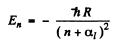we use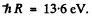Then for n = 2 state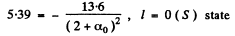α1 = -0.41

for p state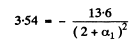α= -0.039

Q.98. Find the Rydberg correction for the 3P term of a Na atom whose first excitation potential is 2.10 V and whose valence electron in the normal 3S state has the binding energy 5.14 eV.

Ans. The energy of the 3p state must be -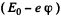where - E0 is the energy of the 3S state.

Then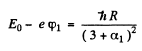so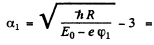Q.99. Find the binding energy of a valence electron in the ground state of a Li atom if the wavelength of the first line of the sharp series is known to be equal to λ1 = 813 nm and the short-wave cutoff wavelength of that series to λ2  = 350 nm.

Ans. For the first line of the sharp series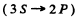in a Li atom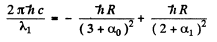For the short wave cut-off wave-length of the same series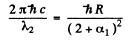From these two equations we get on subtraction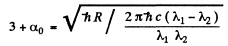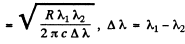Thus in the ground state, the binding energy of the electron is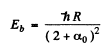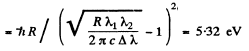Q.100. Determine the wavelengths of spectral lines appearing on transition of excited Li atoms from the state 3S down to the ground state 2S. The Rydberg corrections for the S and P terms are —0.41 and —0.04.

Ans. The energy of the 3 S state is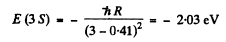The energy of a 2 S state is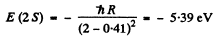The energy of a 2 P state is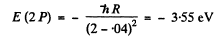We see that

E (2S ) <E (2P)< E ( 3 S)

The transitions are 3S → 2P and 2P →2S.

Direct 3S → 2S transition is forbidden by selection rules. The wavelengths are determined by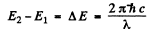Substitution gives

λ = 0.816 μm ( 3S → 2P )

and           λ = 0.674 μm ( 2P → 2S )

Q.101. The wavelengths of the yellow doublet components of the resonance Na line caused by the transition 3P → 3S are equal to 589.00 and 589.56 nm. Find the splitting of the 3P term in eV units.

Ans. The splitting of the Na lines is due to the fine structure splitting of 3 p lines (The 3 s state is nearly single except for possible hyperfine effects.) The splitting of the 3 p level then equals the energy difference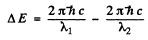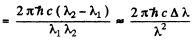Here Δλ = wavelength difference & λ = average wavelength. Substitution gives

ΔE = 2.0 meV

Q.102. The first line of the sharp series of atomic cesium is a doublet with wavelengths 1358.8 and 1469.5 nm. Find the frequency intervals (in rad/s units) between the components of the sequent lines of that series.

Ans. The sharp series arise from the transitions ns → mp . The s lines are unsplit so the splitting is due entirely to the p level. The frequency difference between sequent lines is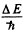and is the same for all lines of the sharp series. It is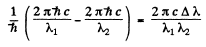Evaluation gives

Q.103. Write the spectral designations of the terms of the hydrogen atom whose electron is in the state with principal quantum number n = 3.

Ans. We shall ignore hyperfine interaction. The state with principal quantum number n = 3 has orbital angular momentum quantum number

/ = 0, 1,2

The levels with these terms are 3 5 , 3 P, 3 D. The total angular momentum is obtained by combining spin and angular momentum. For a single electron this leads to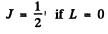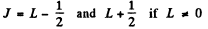We then get the final designations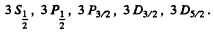Q.104. How many and which values of the quantum number J can an atom possess in the state with quantum numbers S and L equal respectively to
(a) 2 and 3;
(b) 3 and 3;
(c) 5/2 and 2?

Ans. The rule is that if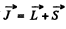then J takes the values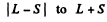in step of 1. Thus :

(a) The values are 1, 2, 3, 4, 5

(b) The values are 0, 1, 2, 3, 4, 5, 6

(c) The values are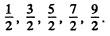Q.105. Find the possible values of total angular momenta of atoms in the states 4P and 6D.

Ans. For the state 4 p, L = 1, S =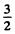( since 2s + 1 = 4 ) . For the state 5 d , Z , = 2 , s = 2 .

The possible values of  J are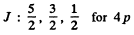The value of the magnitude of angular momentum is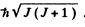Substitution gives the values

4 P :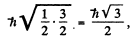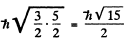and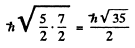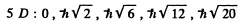Q.106. Find the greatest possible total angular momentum and the corresponding spectral designation of the term
(a) of a Na atom whose valence electron possesses the principal quantum number n = 4;
(b) of an atom with electronic configuration 1s22p3d.

Ans. (a) For the Na atoms the valence electron has principal quantem number n = 4, and the possible values of orbital angular momentum are l = 0 , 1, 2 , 3 so lmax = 3 . The state is 2F, maximum value of J is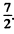Thus the state with maximum angular momentum will be

For this state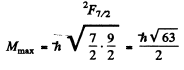(b) For the atom with electronic configuration 1 s2 2 p 3 d . There are two inequivalent valence electrons. The total orbital angular moments will be 1, 2, 3 so we pick l = 3. The total spin angular momentum will be s = 0, 1 so we pick up s = 1. Finally 7 will be 2, 3 , 4 so we pick up 4. Thus maximum angular momentum state is

For this state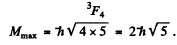Q.107. It is known that in F and D states the number of possible values of the quantum number J is the same and equal to five. Find the spin angular momentum in these states.

Ans. For the f state L = 3 , For the d state L = 2. Now if the state has spin s the possible angular momentum are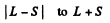The number of J angular momentum values i s 2 S + 1  if L ≥ S and 2 L + 1 if L < S. Since the number of states is 5 , we must have S ≥ L = 2 for D state while S ≤ 3 and 2 S + 1 = 5 in ply S = 2 for F state. Thus for the F state total spin angular momentum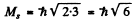while for D state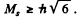Q.108. An atom is in the state whose multiplicity is three and the total angular momentum is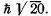What can the corresponding quantum number L be equal to?

Ans. Multiplicity is 2S + l so S = 1 .

Total angular m om entum is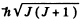so J = 4. Then

L must equal 3, 4, 5

in order that J = 4 may be included in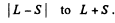Q.109. Find the possible multiplicities x of the terms of the types (a) xD2; (b) xHP3/2; (c) xF1

Ans. (a) Here J = 2, L = 2. Then S = 0, 1 , 2, 3, 4

and the multiplicities( 2S - 1 ) are

1, 3, 5, 7, 9.

(b) Here J = 3 / 2 , L = 1 Then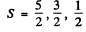and the multiplicities are 6, 4, 2

(c) Here J = 1, L = 3. Then S = 2 , 3 , 4

and the multiplicities are 5, 7, 9

Q.110. A certain atom has three electrons (s, p, and d), in addition to filled shells, and is in a state with the greatest possible total mechanical moment for a given configuration. In the corresponding vector model of the atom find the angle between the spin momentum and the total angular momentum of the given atom.

Ans. The total angular momentum is greatest when L, S are both greatest and add to form J. Now for a triplet of s, p> d electrons Maximum spin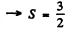corresponding to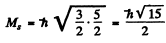Maximum oibital angular momentum     →     L = 3

corresponding to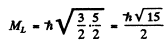Maximum total angular momentum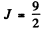corresponding to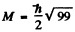In vector model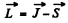or in magnitude squared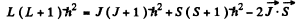Thus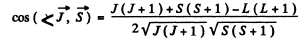Substitution gives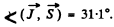Q.111. An atom possessing the total angular momentum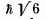is in the state with spin quantum number S = 1. In the corresponding vector model the angle between the spin momentum and the total angular momentum is θ = 73.2°. Write the spectral symbol for the term of that state.

Ans. Total angular momentum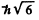means J = 2. It is gives that S = 1.

This means that L = 1,2, or 3 . From vector model relation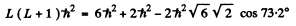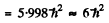Thus E = 2 and the spectral symbol of the state is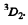Q.112. Write the spectral symbols for the terms of a two-electron system consisting of one p electron and one d electron.

Ans. In a system containing a p electron and a d electron

S = 0,1

L = 1,2,3

For S = 0 we have the terms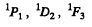For S = 1 we have the terms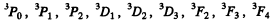Q.113. A system comprises an atom in 2P3/2  state and a d electron. Find the possible spectral terms of that system.

Ans. The atom has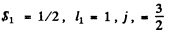The electron has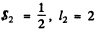so the total angular momentum quantum number must be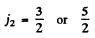In L - S compling we get S = 0, 1 . L = 1 ,2 , 3 and the terms that can be formed are the same as written in the problem above. The possible values of angular momentum are consistant

with the addition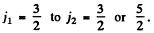The latter gives us                J = 0, 1, 2, 3 ; 1, 2, 3, 4

All these values are reached above.

Q.114. Find out which of the following transitions are forbidden by the selection rules: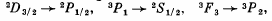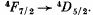Ans. Selection rules are ΔS = 0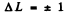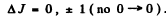Thus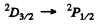is allowed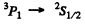not allowed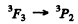is not allowed  (ΔL = 2)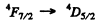is allowed

Q.115. Determine the overall degeneracy of a 3D state of a Li atom. What is the physical meaning of that value?

Ans. For a 3 d state of a Li atom,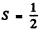because there is only one electron and L = 2.
The total degeneracy is

g = (2L + 1) (2S + 1) = 5 x 2 = 10.

The states are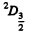and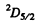and we check that

g = 4 + 6 =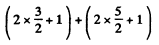Offer running on EduRev: Apply code STAYHOME200 to get INR 200 off on our premium plan EduRev Infinity!

111 docs

,

,

,

,

,

,

,

,

,

,

,

,

,

,

,

,

,

,

,

,

,

;